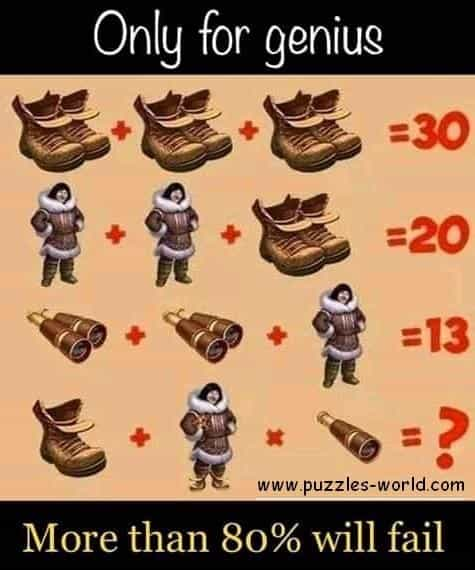# Shoes Man Telescopes puzzle## Shoes Man Telescopes puzzleOnly for genius

Shoes + Shoes + Shoes = 30
Man + Man + Shoes = 20
Telescopes + Telescopes + Man = 13
Shoe + Man x Telescope = ?

More than 80% will fail

The Text above is only for instructional Purposes. To Solve the puzzle, closely observe the image.

There are two shoes in each part of the first question, so there are six shoes altogether, so a single Shoe is worth 5.

There are two Man and two shoes in the second part so a Man is also worth 5.

There are two Telescopes and one Man in the third part, so a telescope is worth 2.

Therefore a Shoe + a Man x Telescope = 5 + 5 x 2 = 15
But here is the catch,
The man(5) is wearing 2 shoes(10) and has held 2 telescopes(4) in the hand.
So the man here is worth 5+10+4 = 19.

Therefore answer will be calculated as
1 shoe + 1 Man x 1 Telescope = 5 + 19 x 2 = 5 + 38 = 43